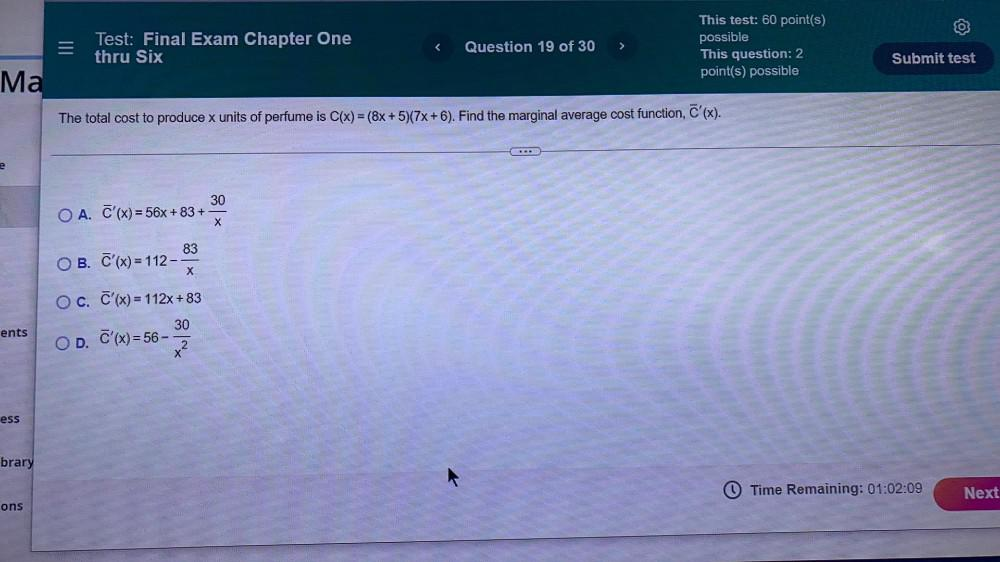Question:

# The total cost to produce x units of perfume is C(x) = (8x + 5)(7x+6). Find the marginal average cost function, C'(x). ○ A. C'(x) = 56x+83+30/x ○ B. C'(x) = 112-83/x ○ C. C'(x)= 112x + 83 ○ D. C'(x)=The total cost to produce x units of perfume is C(x) = (8x + 5)(7x+6). Find the marginal average cost function, C'(x). ○ A. C'(x) = 56x+83+30/x ○ B. C'(x) = 112-83/x ○ C. C'(x)= 112x + 83 ○ D. C'(x)=56-30/x²